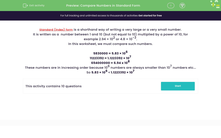# Compare Numbers in Standard Form

In this worksheet, students will compare numbers written in standard form or as normal numbers.Key stage:  KS 3

Curriculum topic:   Number

Curriculum subtopic:   Interpret/Compare Standard Form Numbers

Difficulty level:#### Worksheet Overview

Standard (index) form is a shorthand way of writing a very large or a very small number.

It is written as a  number between 1 and 10 (but not equal to 10) multiplied by a power of 10, for example 2.94 × 102 or 4.8 × 10-3.

In this activity, we will compare such numbers.

5830000 = 5.83 × 106

11223392 = 1.1223392 × 107

694000000 = 6.94 x 108

These numbers are in increasing order because 106  numbers are always smaller than 107 numbers etc...

So,  5.83 × 106 < 1.1223392 × 107

Very small numbers can be written in standard form too and these will have negative powers.

0.00574 = 5.74 x 10-3

0.00069 = 6.9 x 10-4

0.000028 = 2.8 x 10-5

These numbers are getting smaller because 10-5  numbers are always smaller than 10-4  numbers.

So,  2.8 x 10-5 <  6.9 x 10-4

Let's have a go at comparing some numbers in standard form.

### What is EdPlace?

We're your National Curriculum aligned online education content provider helping each child succeed in English, maths and science from year 1 to GCSE. With an EdPlace account you’ll be able to track and measure progress, helping each child achieve their best. We build confidence and attainment by personalising each child’s learning at a level that suits them.

Get started